Latest SSC jobs   »   Area of Triangle

# Area of Triangle, Formulas With Examples

## Area of Triangle

Area of Triangle: Formulas With Examples are provided in this post. A triangle is a polygon, a 2-dimensional object with 3 sides and 3 vertexes. The area of triangular shapes is determined by using a simple formula to be used while solving problems or questions. You must know the length of the sides, the type of triangle, and the height of the triangle in order to find the area of a triangle. What is the formula to determine the area of Triangles? This post will answer all your queries related to the area of triangles.

Get free notes on Maths

### Area of Triangle: Definition & Types of Triangle

A triangle is a two-dimensional polygon having 3 sides and 3 angles. An area of a triangle is the region occupied inside the triangle. A triangle can be of 4 types depending upon the length of its sides or angles. The 4 types of triangles are:

1. Right Angled Triangle- In which one angle is 90 degrees.
2. Isosceles Triangle- In which 2 sides are equal.
3. Equilateral Triangle- In which all the three sides are equal and hence each angle is of 60°
4. Scalene triangle- In which all the three sides are unequal.

## Triangle Formula

The general formula for the area of a triangle is given by 1/2 x Base x Perpendicular. The area will depend upon the type of triangle. Let’s take a look at the formula to find the area for each type of triangle.

### Area of Right Angled Triangle Formula

A right-angled triangle is a triangle in which one angle is 90°. The relation between the sides and angles of a right-angled triangle is the basis for trigonometry. The side opposite the right angle is the hypotenuse. The formula to determine the area of the right-angled triangle is given below.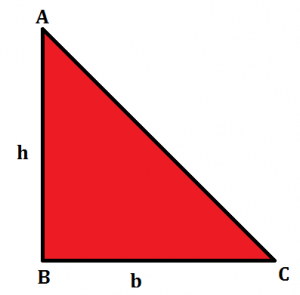In the right-angled triangle given, we have the perpendicular as ‘h’ and base as ‘b’ So the formula for the area of a right-angled triangle can be given by:

### Area of Isosceles Triangle Formula

In an isosceles triangle, you will have 2 sides of equal lengths. Hence, the 2 angles of this triangle will be equal to one another. In the figure given below, we have an isosceles triangle with two equal sides, ‘a’ and the base as ‘b’. A perpendicular is drawn from A to D which divides the base into 2 equal parts.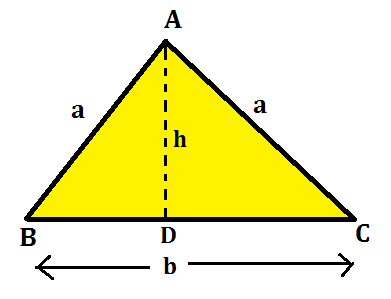Hence, by the formula, A = 1/2 x b x h, we can derive the formula for the area of the isosceles triangle by the formula given below:

### Area of Equilateral Triangle

In an equilateral triangle, you will have the 3 sides of equal lengths. Hence, all the 3 angles of this triangle will be equal and of 60 degrees. In the figure given below, we have an equilateral triangle with equal sides as ‘a’. A perpendicular is drawn from A to D which divides the base into 2 equal parts.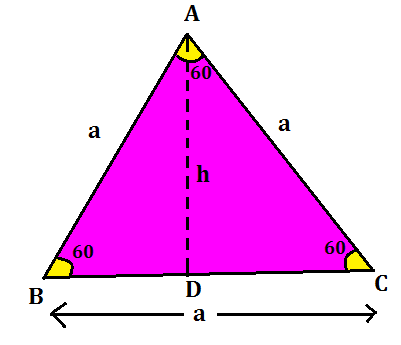Hence, by the formula, A = 1/2 x b x h, the formula for the area of the equilateral triangle can be derived as:

### Area of a triangle by Heron’s Formula

A scalene triangle is a triangle in which all the 3 sides are unequal and no angle is 90 degrees. To find the area of a scalene triangle and even other triangles, we use Heron’s Formula. The formula given by Heron about the area of a triangle is also known as Hero’s formula.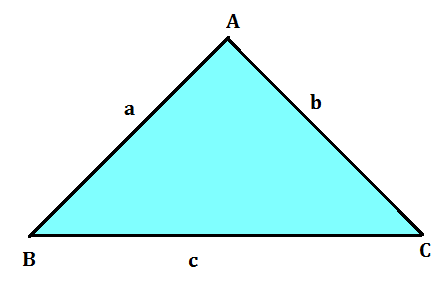From the figure given above, a scalene triangle is given with 3 sides as ‘a’, ‘b’, and ‘c’. Heron’s formula is stated as: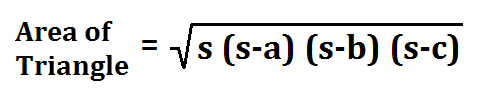where a, b and c are the sides of the triangle, and s = semi-perimeter, i.e., half the perimeter of the triangle. The semi-perimeter of the triangle is given by: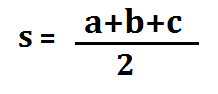### Area of Triangle: Examples

Example 1: Find the area of a triangle, two sides of which are 8 cm and 11 cm and the perimeter is 32 cm.

Solution: Here we have perimeter of the triangle = 32 cm, a = 8 cm and b = 11 cm.
Third side, c = 32 cm – (8 + 11) cm = 13 cm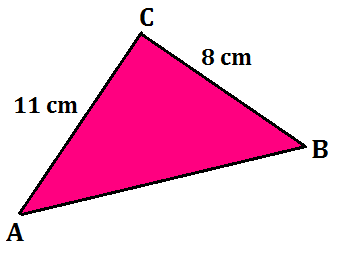So, 2s = 32, i.e., s = 16 cm,
s – a = (16 – 8) cm = 8 cm,
s – b = (16 – 11) cm = 5 cm,
s – c = (16 – 13) cm = 3 cm.

Therefore, the area of the triangle =
√16 x 8 x 5 x 3 = 8√30 cm²

Example 2: Find the area of a right-angled triangle with a base of 14 cm and a height of 5 cm.

Solution: Area of a right-angled triangle = 1/2 x b x h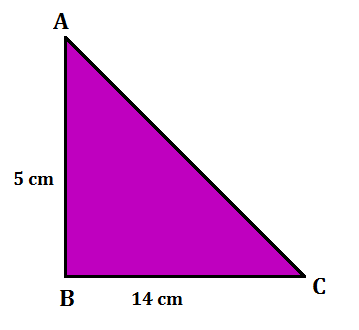Hence, the area of the right-angled triangle is

A= 1/2 x 14 x 5 = 35 cm²

## Area of Triangle: FAQ

Q. What is the area of the triangle formula?

Ans: The basic formula to find the area of a triangle is, area of triangle = 1/2 (b × h); where ‘b’ is the base and ‘h’ is the height of the triangle.

Q. What are the three sides of a triangle?

Ans: In a right triangle, the hypotenuse is the longest side, an “opposite” side is the one across from a given angle, and an “adjacent” side is next to a given angle.

Q. How do you find the area of a triangle?

Ans: The area of a triangle is calculated by using it is equal to half of the base times height, i.e. A = 1/2 × b × h.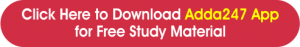You may also like to read:

#### Congratulations!General Awareness & Science Capsule PDFIncorrect details? Fill the form again here

General Awareness & Science Capsule PDF

Thank You, Your details have been submitted we will get back to you.
•SSC CPO Salary: Job Profile, Allowances,...
•Independence Day 2022, History & Cel...
•Happy Raksha Bandhan!!
•India's Biggest Govt Exams Book Fair, Fl...
•Merchant Navy Salary in India, Job Profi...
•10 Tips to Crack SSC CGL Exam: SSC CGL P...